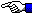# The Pythagorean Theorem

`M. Gallant 1/99`
``` The Pythagorean Theorem is probably the best known theorem of basic geometry: The square of the hypotenuse of a right-angle triangle is equal to the sum of the squares of the other two sides Euclid in his Elements provided a somewhat complicated proof. Many simpler proofs have been given since Euclid. The Pythagorean Proposition, E.S. Loomis 1940 provides 370 proofs of this theorem! The following two Java applets demonstrate two of the better known geometrical proofs from antiquity of the Pythagorean Theorem:    References: Mathematical Recreations and Essays, W. W. Rouse Ball and H. S. M Coxeter, 13th Edn. Dover 1987, p. 88. A Bibliography of Recreational Mathematics, W. L. Schaaf, V 4, National Council of Teachers of Mathematics, p. 75. Dissections: Plane and Fancy, G. Frederickson, Cambridge University Press, 1997.Go Home ET ```